# ML Aggarwal Solutions for Class 6 Maths Chapter 3 Integers

ML Aggarwal Solutions for Class 6 Maths Chapter 3 Integers provides clear knowledge of fundamental concepts discussed in this Chapter. The solutions can be referred by the students to enhance their problem solving and time management skills. Practising these solutions on a regular basis, helps them to come out with flying colours in the final exams. In order to obtain more knowledge, students can refer to ML Aggarwal Solutions for Class 6 Maths Chapter 3 Integers PDF, from the links which are available below

Chapter 3 explains important concepts based on Integers. The solutions are designed in a stepwise manner to make learning fun for the students. These solutions help them to obtain an idea of solving difficult problems in a shorter duration effortlessly.

## ML Aggarwal Solutions for Class 6 Maths Chapter 3 Integers Download PDF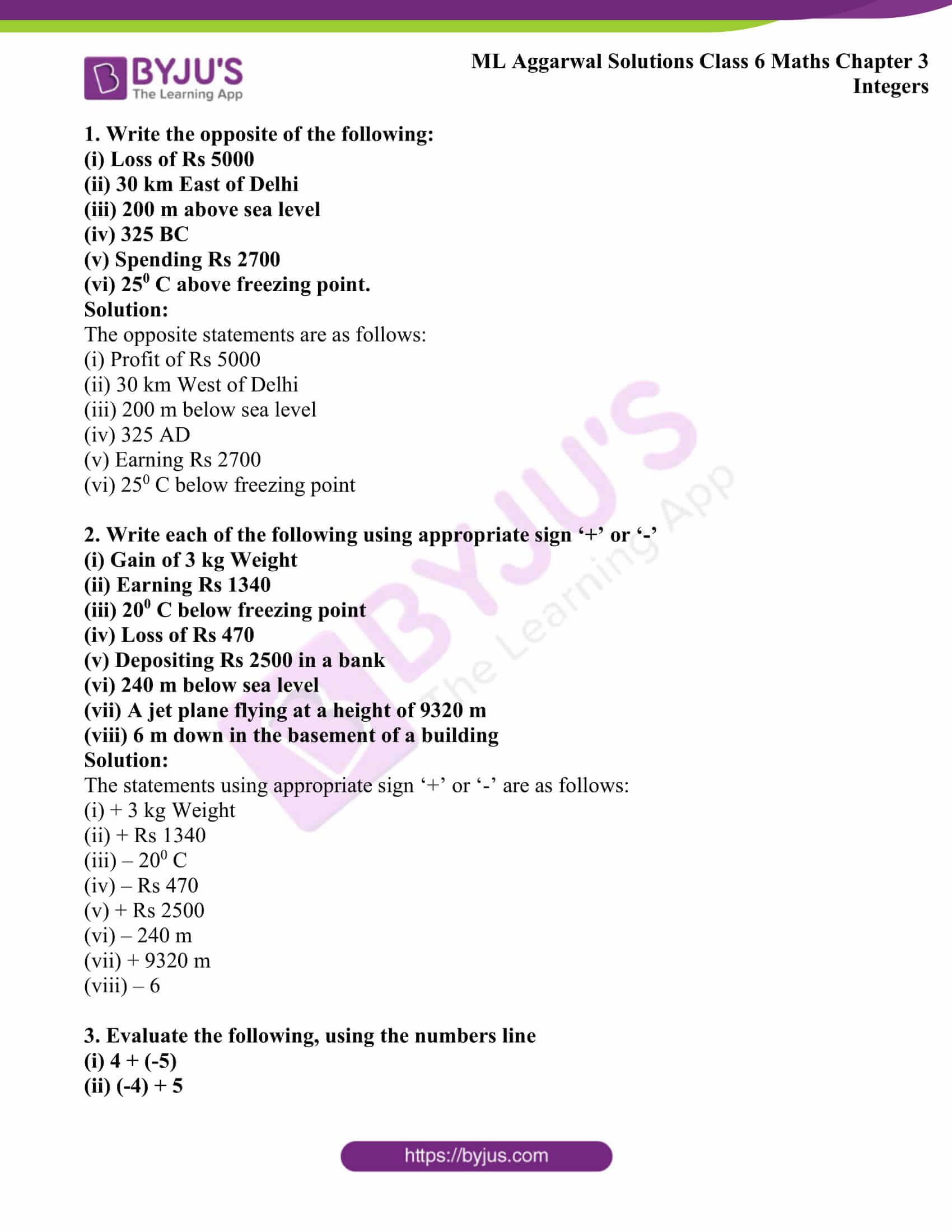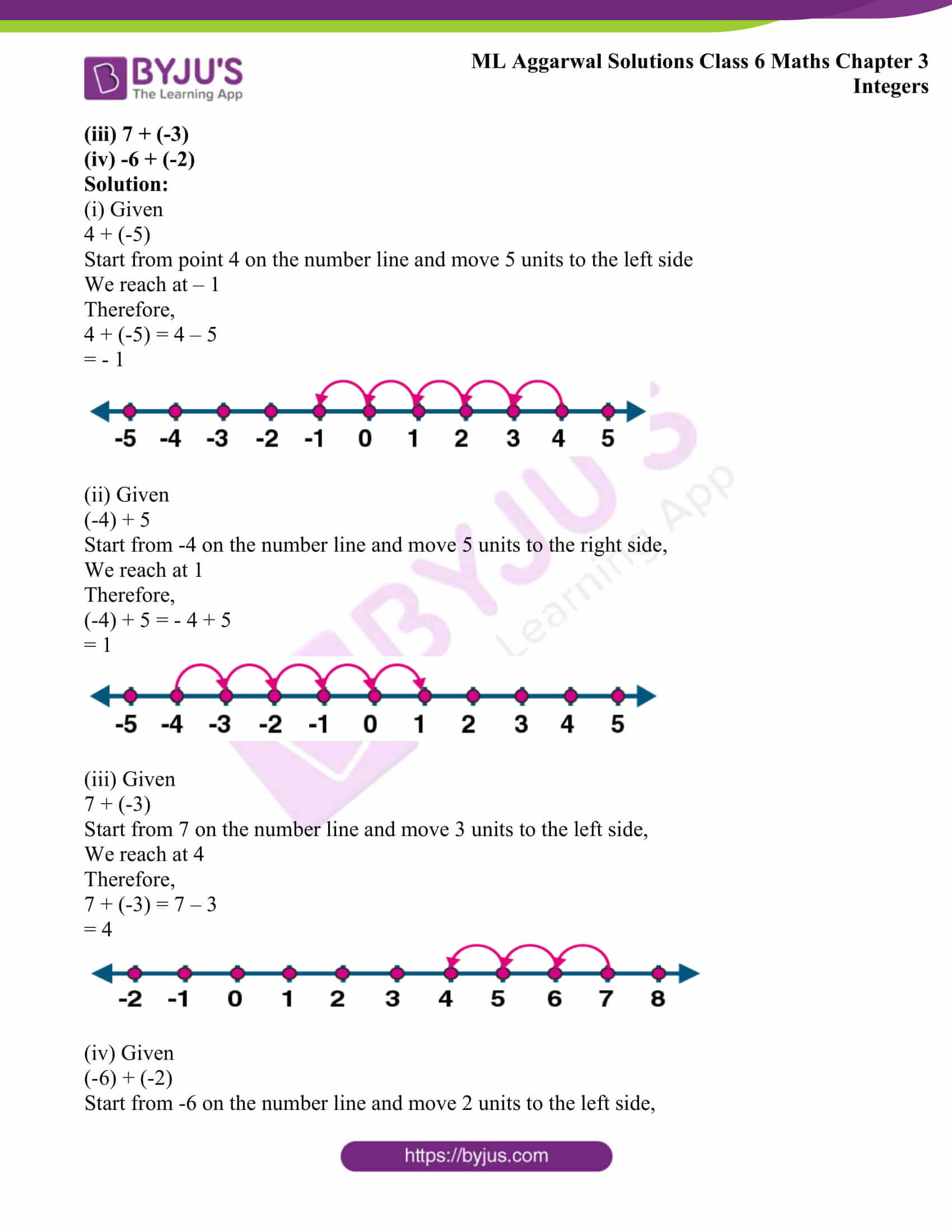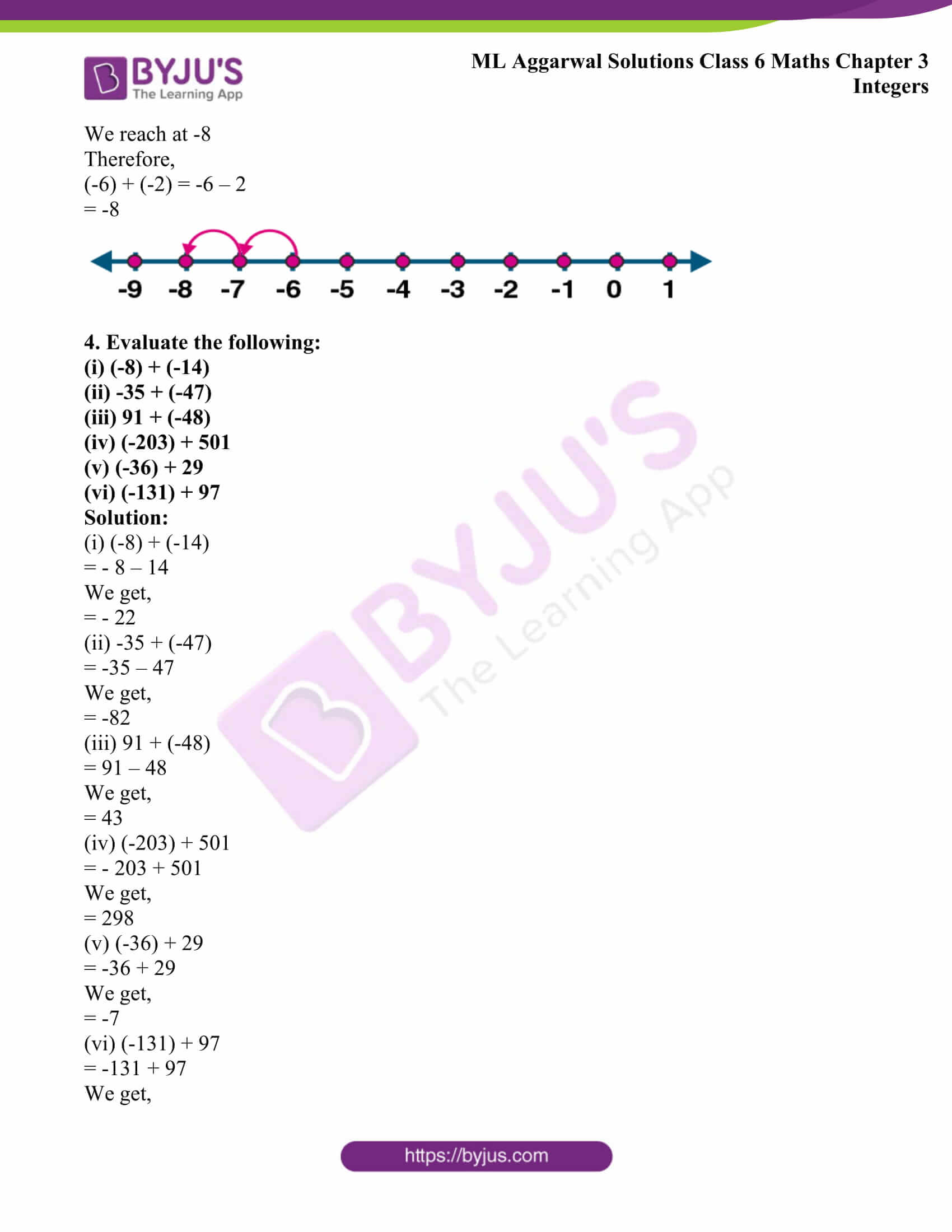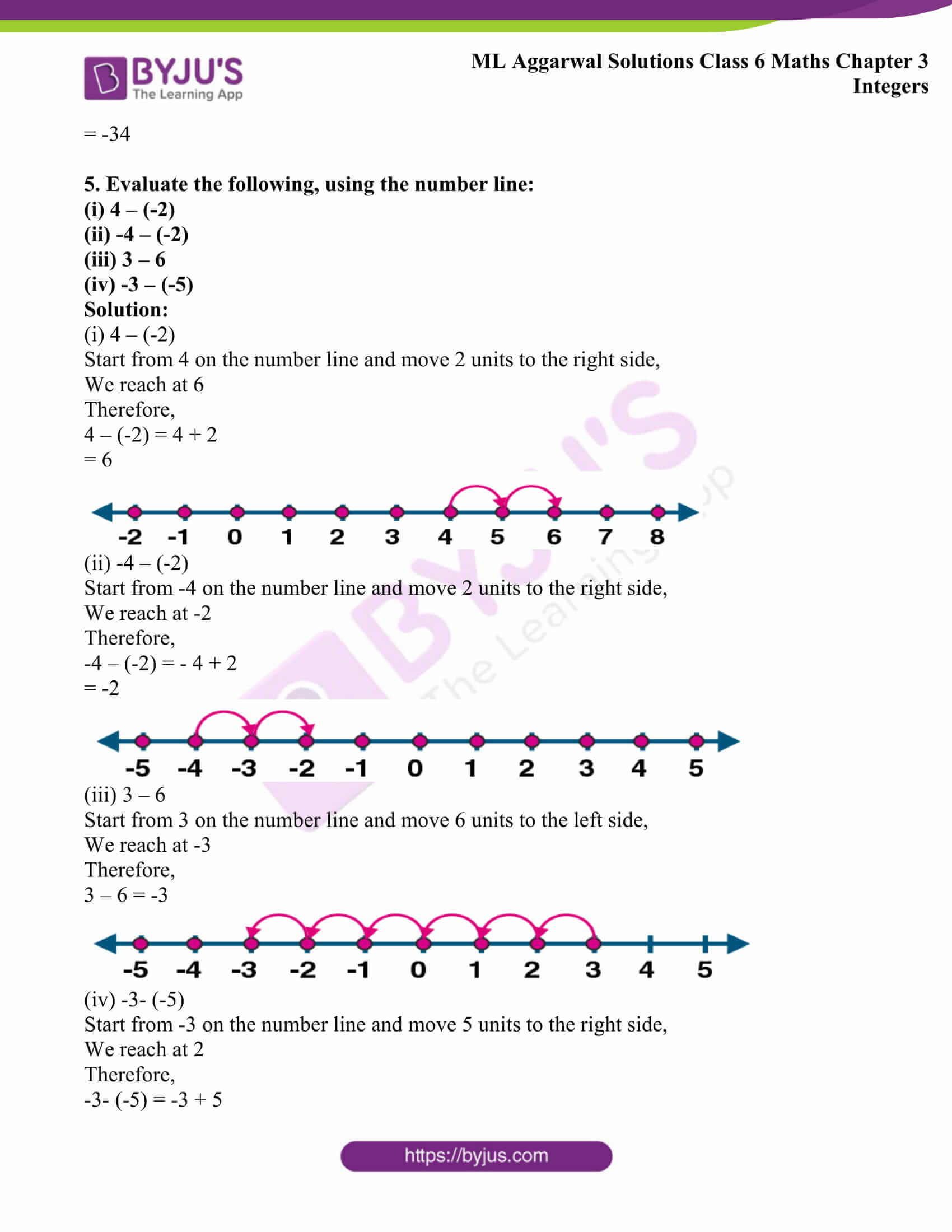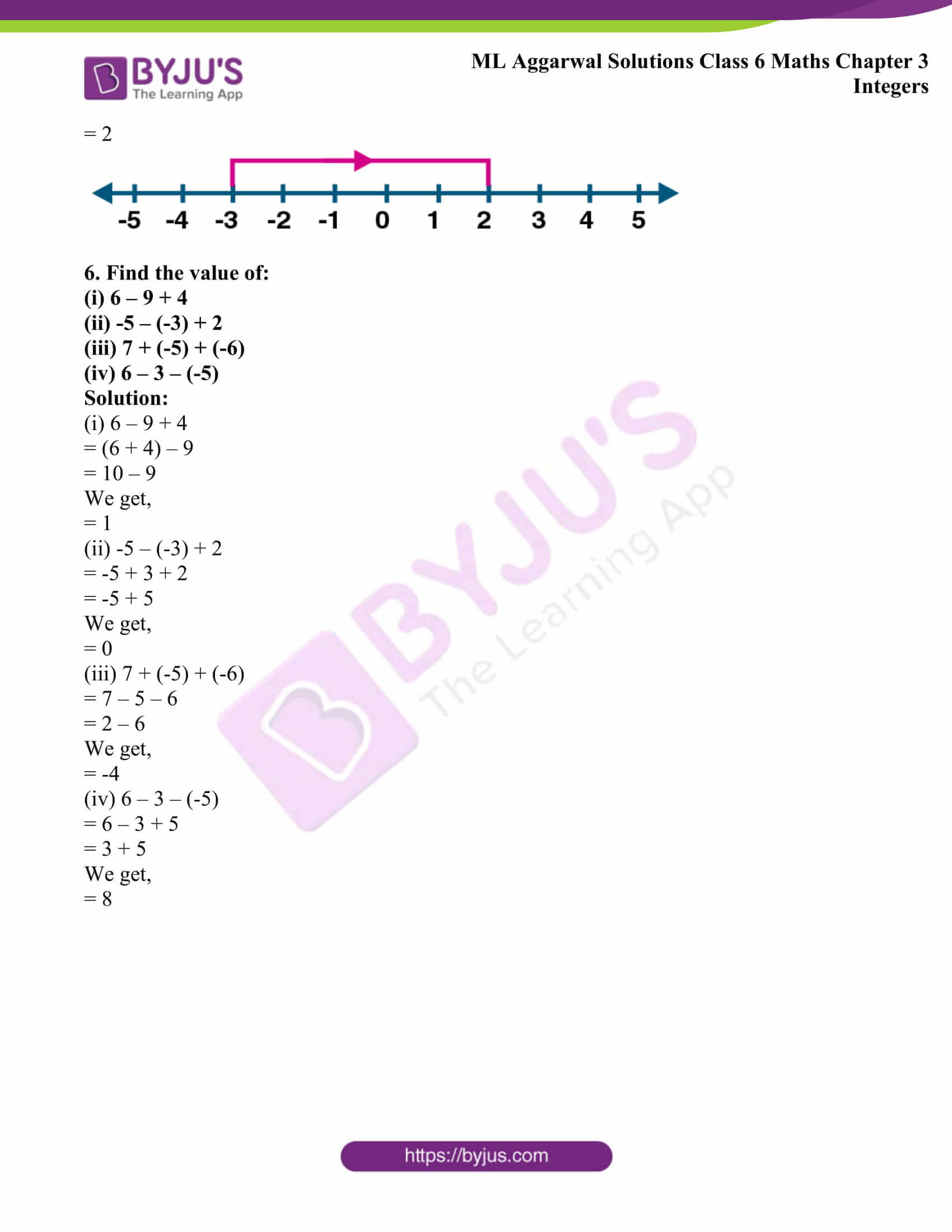## Access answers to ML Aggarwal Solutions for Class 6 Maths Chapter 3 Integers

1. Write the opposite of the following:

(i) Loss of Rs 5000

(ii) 30 km East of Delhi

(iii) 200 m above sea level

(iv) 325 BC

(v) Spending Rs 2700

(vi) 250 C above freezing point.

Solution:

The opposite statements are as follows:

(i) Profit of Rs 5000

(ii) 30 km West of Delhi

(iii) 200 m below sea level

(v) Earning Rs 2700

(vi) 250 C below freezing point

2. Write each of the following using appropriate sign ‘+’ or ‘-’

(i) Gain of 3 kg Weight

(ii) Earning Rs 1340

(iii) 200 C below freezing point

(iv) Loss of Rs 470

(v) Depositing Rs 2500 in a bank

(vi) 240 m below sea level

(vii) A jet plane flying at a height of 9320 m

(viii) 6 m down in the basement of a building

Solution:

The statements using appropriate sign ‘+’ or ‘-’ are as follows:

(i) + 3 kg Weight

(ii) + Rs 1340

(iii) – 200 C

(iv) – Rs 470

(v) + Rs 2500

(vi) – 240 m

(vii) + 9320 m

(viii) – 6

3. Evaluate the following, using the numbers line

(i) 4 + (-5)

(ii) (-4) + 5

(iii) 7 + (-3)

(iv) -6 + (-2)

Solution:

(i) Given

4 + (-5)

Start from point 4 on the number line and move 5 units to the left side

We reach at – 1

Therefore,

4 + (-5) = 4 – 5

= – 1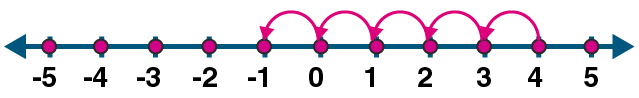(ii) Given

(-4) + 5

Start from -4 on the number line and move 5 units to the right side,

We reach at 1

Therefore,

(-4) + 5 = – 4 + 5

= 1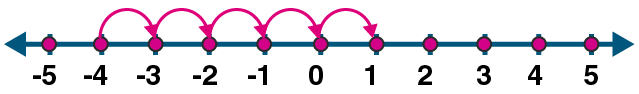(iii) Given

7 + (-3)

Start from 7 on the number line and move 3 units to the left side,

We reach at 4

Therefore,

7 + (-3) = 7 – 3

= 4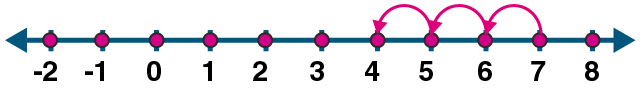(iv) Given

(-6) + (-2)

Start from -6 on the number line and move 2 units to the left side,

We reach at -8

Therefore,

(-6) + (-2) = -6 – 2

= -8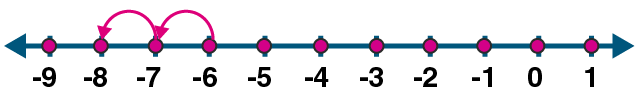4. Evaluate the following:

(i) (-8) + (-14)

(ii) -35 + (-47)

(iii) 91 + (-48)

(iv) (-203) + 501

(v) (-36) + 29

(vi) (-131) + 97

Solution:

(i) (-8) + (-14)

= – 8 – 14

We get,

= – 22

(ii) -35 + (-47)

= -35 – 47

We get,

= -82

(iii) 91 + (-48)

= 91 – 48

We get,

= 43

(iv) (-203) + 501

= – 203 + 501

We get,

= 298

(v) (-36) + 29

= -36 + 29

We get,

= -7

(vi) (-131) + 97

= -131 + 97

We get,

= -34

5. Evaluate the following, using the number line:

(i) 4 – (-2)

(ii) -4 – (-2)

(iii) 3 – 6

(iv) -3 – (-5)

Solution:

(i) 4 – (-2)

Start from 4 on the number line and move 2 units to the right side,

We reach at 6

Therefore,

4 – (-2) = 4 + 2

= 6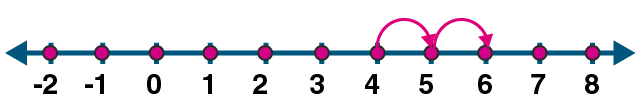(ii) -4 – (-2)

Start from -4 on the number line and move 2 units to the right side,

We reach at -2

Therefore,

-4 – (-2) = – 4 + 2

= -2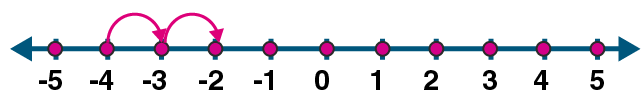(iii) 3 – 6

Start from 3 on the number line and move 6 units to the left side,

We reach at -3

Therefore,

3 – 6 = -3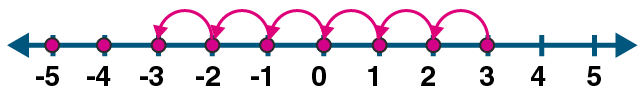(iv) -3- (-5)

Start from -3 on the number line and move 5 units to the right side,

We reach at 2

Therefore,

-3- (-5) = -3 + 5

= 2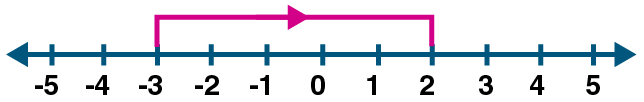6. Find the value of:

(i) 6 – 9 + 4

(ii) -5 – (-3) + 2

(iii) 7 + (-5) + (-6)

(iv) 6 – 3 – (-5)

Solution:

(i) 6 – 9 + 4

= (6 + 4) – 9

= 10 – 9

We get,

= 1

(ii) -5 – (-3) + 2

= -5 + 3 + 2

= -5 + 5

We get,

= 0

(iii) 7 + (-5) + (-6)

= 7 – 5 – 6

= 2 – 6

We get,

= -4

(iv) 6 – 3 – (-5)

= 6 – 3 + 5

= 3 + 5

We get,

= 8